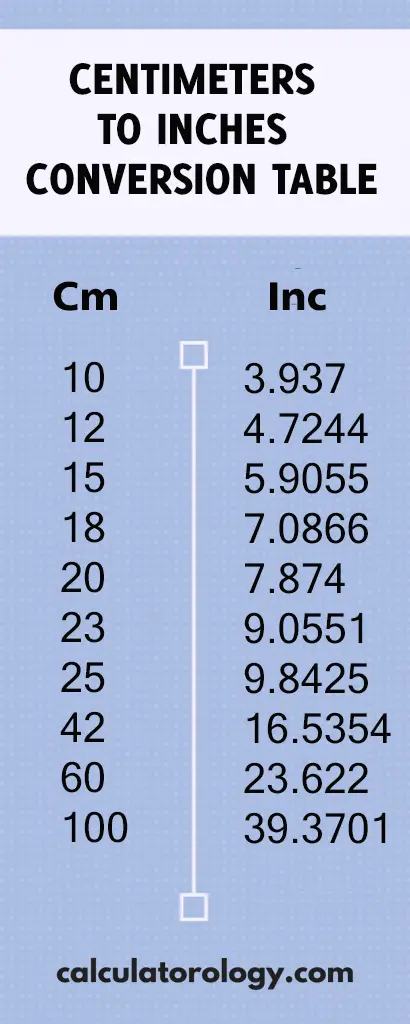## 9.842519685 inches

### 25 cm to inches

This conversion calculator is used to convert the value in centimeters (cm) to the value in inches (in). It uses a simple formula to execute the calculations and also gives accurate results based on the input. When using the converter, the first step is to enter the value to be converted in the blank text field. The ‘Convert’ button initiates the conversion from centimeters to inches.

To convert 25 cm into inches, it is important to determine the number of inches that make up one centimeter.

#### How many inches in one centimeter?

1 cm = (1/2.54)” = 0.3937007874

This means that there are 0.3937007874 inches in one centimeter.

D (in) = D (cm) / 2.54

The distance in inches is determined by dividing the value in centimeters by 2.54.## 25cm to Inches Conversion

 cm inches

#### How to convert 25cm to inches

Since 1 centimeter is equivalent to 0.3937007874 inches, how many inches are there in 25 centimeters?

If 1 cm = 0.3937007874 inches,

25cm =? Inches

Calculation:     25cm / 2.54

= 9.842519685"

Inches fraction result:  9 27/32

Feet+inches result:       0ft 9.842519685

This implies that there are 9.842519685inches in 25 centimeters.

The conversion calculator can be used to perform other conversions using different values. The centimeter to inches conversion calculator can only execute a single conversion at a time. The ‘Reset’ button is used to erase all information of the previous calculations to create a new platform for more conversions.

25 cm to inches can also be determined using the Centimeter to Inches conversion table. It gives the conversion results of centimeters to inches based on a range of 0.01cm to 100cm.

##### Definitions

A centimeter is a length unit in the metric system abbreviated as "cm". One centimeter is equal to 0.3937007874 inches.

An inch is a US customary and imperial measurement system that is used widely and is abbreviated as (in) or using the symbol (“) - double prime.